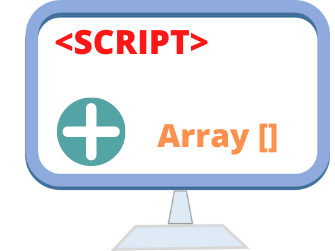# Total number of elements in an array using length propertyWe can find the length or size of any JavaScript array by using its length property.
``array.length``
To access the elements of the array we can use this length property to loop through. Please note that first element of an array starts from 0 so array_name is the second element of the array where as array_name is the first element of the array. Same way the last element of the array is the total size of the array minus one. We will see example of this here.

Let us start with a simple array consisting of some scripting languages ( or any thing of your choice )
``````
<script type="text/javascript">
var scripts = new Array();
scripts = "PHP";
scripts = "ASP";
scripts = "JavaScript";
scripts = "HTML";

document.write(scripts.length);
</script>``````
Output of above code is 4

## Displaying element using length

We will add one line to above to display an element of the array.
``````
<script type="text/javascript">
var scripts = new Array();
scripts = "PHP";
scripts = "ASP";
scripts = "JavaScript";
scripts = "HTML";

document.write(scripts.length);
document.write(scripts[scripts.length]);
</script>``````
You can see the above code will display error message saying undefined. Why ?
The reason is we are trying to display beyond the last element of the array. So the last element of the array can be accessed by not (scripts.length) but by (scripts.lenght -1)

So the correct code is here to display the last element of an array

``````
<script type="text/javascript">
var scripts = new Array();
scripts = "PHP";
scripts = "ASP";
scripts = "JavaScript";
scripts = "HTML";
document.write(scripts[scripts.length-1]);
</script>``````
This will display HTML as last element of the array

## Assigning Array length property value

What happens when we assign a value to length property of the array which is less than the actual size ( or length ) of the array?

The elements after the assigned length are lost!

Let us see this example where after storing four elements in the array we will limit the length value of the array to 2 and see what happens.
``````<script type="text/javascript">
var scripts = new Array();
scripts = "PHP";
scripts = "ASP";
scripts = "JavaScript";
scripts = "HTML";

document.write(scripts[scripts.length-1]); // Print HTML
scripts.length=2;
document.write("<br>"); // One line break
document.write(scripts[scripts.length-1]); // Print ASP

</script>``````
We lost last two elements of the array by assigning less value to the array length property.

## How many words present in a string

We will use one string and then create an array by using split function. Then by using length property we can find out how many words are present. Here is the example
``````<script type="text/javascript">
var str="Welcome to plus2net. You can learn web programming and use them in your applications";
var a1 = new Array();
a1=str.split(" ");
document.write("total Number of words present: "+ a1.length);
</script>``````
Output is here
``total Number of words present: 14``## Subscribe

* indicates required
Subscribe to plus2netplus2net.com

 Gabriel 09-03-2013 How do you get this to work?